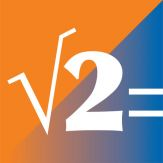\$9.99

# iPhone Giveaway of the Day - SciPro Math

(107 votes)
"SciPro Math" is an extraordinary scientific calculator, designed for portable devices
\$9.99 本日 無料提供

SciPro Math
は今日のGiveawayとして利用可能です!"SciPro Math" is an extraordinary scientific calculator, designed for portable devices, that contains hundreds of mathematical operations and has a great capability to store data. It can be used for doing unit conversions, algebra, geometry, trigonometry, pre-calculus, probability, and finance. It has been designed for both students and professionals.

FOR TUTORIALS, GO TO:
https://youtube/SciPro Math

BENEFITS:
Using storage variables to hold and store the values of the coefficients, you are now able to enter entire linear, quadratic, cubic, and even quartic equations right on the application itself, and save, solve, and evaluate them, live.

There is no need to write that much. Just solve and store the solutions right on the calculator. It has a great storage capability available for you to use.

Use the calculator for doing unit conversions within a particular system of measurements, but also for performming unit conversions across different systems, namely: the imperial and metric.

Instead of doing complex calculations by hand, like evaluating equations, avoid making silly mistakes, let "SciPro Math" do them for you.

Since the calculator can simulate tossing a coin or rolling a dice, it is a very useful tool to have next to you for playing games of chance.

TOPICS:
"SciPro Math" is the perfect companion for your mathematics class. Consider it for the following topics and subjects:
• Arithmetic
• Conversions
• Algebra
• Linear Algebra
• Geometry
•  Trigonometry
•  Probability
• Precalculus
• Finance

FEATURES:
•  Contains 16 different screens of operations
•  Performs over 400 OPERATIONS
•  Calculations are displayed on the screen
• Each screen of operations has a unique color scheme
• Pressed buttons light up
• Highlight colors
• A color management system
•  Memory variables: Av to Zv
•  A Value Transfer Function “->(X)n” that is used to store numbers in the application
•  Simple operation display
•  Save program function
• Load program function
• Color scheme switch
•  2x2 and 3x3 matrices
•  Reset function
•  Delete key
• All-clear key
•  Formatted calculations
• Navigation system
• 4 navigation functions
• 2nd, 3rd, rad (4th), and 5th functions
• The navigation system manages the operations and the colors displayed on each of the buttons

### 開発者:

Roberto Antonio Campusano Acosta

Utilities

2.0

17.93 MB

4+

English

### 互換性:

iPhone, iPad, iPod touch

## コメント SciPro Math

Windows Giveaway of the Day\$25.95

ご必携のツールSD/HD/4K FLV動画像処理